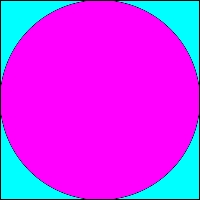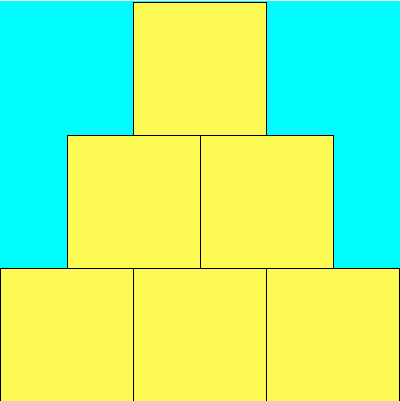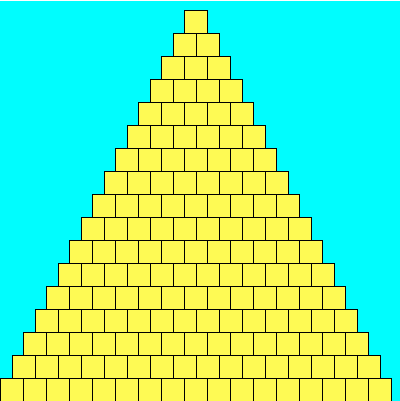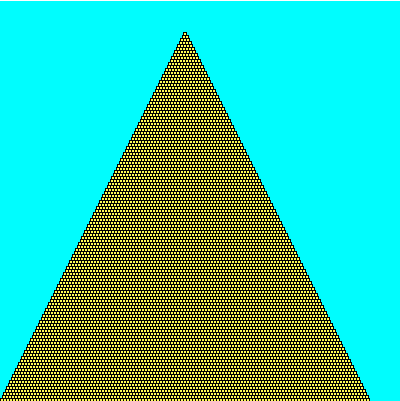# PreLab 03

Due in class on Wednesday, February 17

In this prelab you will formulate some of the mathematical ideas necessary to complete Lab 03. Note that there are 5 problems, numbered 1 through 5.

## Part 0 - Practice With Conditionals

1. Consider the following code snippet:
```	for n in [10, 9, 6, 5]:		if (n%2) == 0 :			print( n )		else:			print( n*2 )			if (n%3) == 0 :				print( n/3 )

```
What will this print?

## Part 1 - Monte Carlo!

You probably remember bumping into that peculiar number π = 3.14159265..., right? It comes up when you're talking about circles and trigonometry, but also appears in a bunch unexpected places that seem to have little to do with either. As a refresher, Pi can be defined as the ratio of a circle's circumference (π d) to its diameter (d). One interesting feature of Pi is that it's an irrational number, meaning it cannot be expressed as a fraction m/n where both m and n are integers; consequently, its decimal representation never ends or even repeats.

Since ancient times, mathematicians have been fascinated with the study of Pi and it's various properties. Early approximations of Pi, such as 22/7 and 355/113 were accurate to 3 and 7 digits repsectively (the latter approximation was the best known for nearly a millenium). Currently, more than the first trillion (a million million) digits are known. There are many ways to estimate Pi -- for example, you could draw as precise a circle as you can manage, measure its circumference C and diameter d, and then divide C/d; this should give you Pi. Alternatively, there is a geometry-based approach due to Archimedes. We'll investigate a third approach using what is called a Monte Carlo method.

### Monte Carlo Method

When we say we're using a Monte Carlo method, we usually mean we're going to do a bunch of random trials and observe the fraction of those trials that have a certain property. In our case, we're going to be throwing darts into a square region, and computing the fraction of those darts that land within a circle inscribed inside that square. Each throw is a trial, and the property we are concerned with is whether or not the dart landed inside the circle or not.

Describe the Problem:
The problem you will solve on your lab is as follows.
input: get a number of trials n from the user.
goal: compute and output an approximation to Pi using a Monte Carlo method with n trials.

Understand the Problem:
More precisely, we'll begin by (theoretically) constructing a target circle inscribed in a square. This is our dart board, and the target circle reaches all the way to the edge of the square. It might look something like the following:Next, we'll simulate repeatedly throwing darts at random against the board above (we'll assume that our random throws alway hits the square, and are equally likely to land at any given point inside the square). We then look at the fraction of the darts that land within the circle out of all those that were thrown. I claim that if we then multiply this fraction by 4, we should have a good approximation to Pi. What sort of dark sorcery is this? Let's take a closer look.

Suppose you are throwing darts at the square board that has a figure drawn on it. Assuming the dart lands on the board, the chance that it is inside the figure is the ratio of the area of the figure to the area of the square. So in the picture above the ratio of the area of the circle to the area of the square is the probability that a dart hits the circle, and this probability can be estimated by the number of darts that hit the circle divided by the number of darts that hit the square..

2. Suppose the circle has a radius of r.
• What is the length of one side of the square board in terms of r?
• What is the area of the square board in terms of r?
• What is the area of the inscribed circle in terms of r?
• Use these to determine the probability that a randomly thrown dart lands within the circle. That is, determine the ratio R of the two areas (the circle's area divided by that of the square). Simplify your expression for R (it will have a Pi in it). Then give an expression for Pi in terms of R using (simple) algebraic manipulation.

## Part 2 - Walk Like an Egyptian

Describe the Problem:
The problem you will solve on your lab is as follows.
input: get a numbers width from the user, as well as the number of bricks tall to make the pyramid (n).
goal: draw a pyramid that is n bricks tall in a square canvas width wide (and thus width tall).

Understand the Problem:
Here are three sample outputs for your reference. Notice that the pyramid doesn't necessarily fill the entire canvas to the right and to the top; if the canvas width is not evenly divisible by the number of bricks, then there will be extra blank space. (A question for you to ponder: why is there so much blank space in the third example? Seems like you could fit lots of extra bricks both to the right and up top...)

400 x 400, 3 bricks.400 x 400, 17 bricks.400 x 400, 123 bricks.3.Notice that our bricks are square and our canvas is square. If the canvas is width by width pixels and the pyramid is n bricks tall, what is the size in pixels of each brick?

4. Suppose the canavs is 600 by 600. The horizontal coordinates on the canvas go from 0 on the left edge to 600 on the right. The vertical coordinates go from 0 at the top to 600 at the bottom. Draw a copy of the first pyramid, which has 3 bricks at the base and is 3 bricks tall. Label the coordinates of the top left corner of each brick. Keep this in front of you as you answer the next question.

5. Suppose width is the size of the canvas and s is the size of one brick. Let rowX and rowY be the coordinates of the upper left corner of the first (leftmost) brick in each row.

1. What are rowX and rowY for the bottom row of the pyramid?
2. Let x and y be the coordinates of the upper left corner of a brick as we walk along one row of the pyramid. Of course, these start out as rowX and rowY. How do x and y change as we go from one brick to the next along each row?
3. How do rowX and rowY change as we move from one row to the row above?

Your answers to question 5 should help you design a nested for-loop to draw the pyramid in the pyramid.py program in part 2 of the lab.

## Honor Code

If you followed the Honor Code in this assignment, write the following sentence attesting to the fact at the top of your homework.

I affirm that I have adhered to the Honor Code in this assignment.